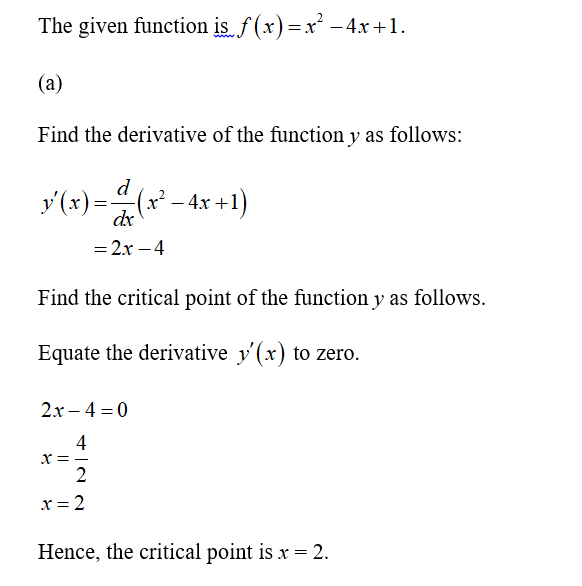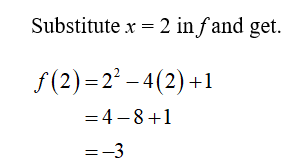# Let f (x) = x2 − 4x + 1. (a) Find the critical point c of f and compute f (c). (b) Compute the value of f (x) at the endpoints of the interval [0, 4]. (c) Determine the min and max of f on [0, 4]. (d) Find the extreme values of f on [0, 1].

Question
1 views

Let f (x) = x2 − 4x + 1.
(a) Find the critical point c of f and compute f (c).
(b) Compute the value of f (x) at the endpoints of the interval [0, 4].
(c) Determine the min and max of f on [0, 4].
(d) Find the extreme values of f on [0, 1].

check_circle

Step 1

Hey, since there are multiple sub-parts posted, we will answer first three sub-parts. If you want any specific sub-part to be answered then please submit that question and specify the sub-part number in your message.

Step 2Step 3...

### Want to see the full answer?

See Solution

#### Want to see this answer and more?

Solutions are written by subject experts who are available 24/7. Questions are typically answered within 1 hour.*

See Solution
*Response times may vary by subject and question.
Tagged in